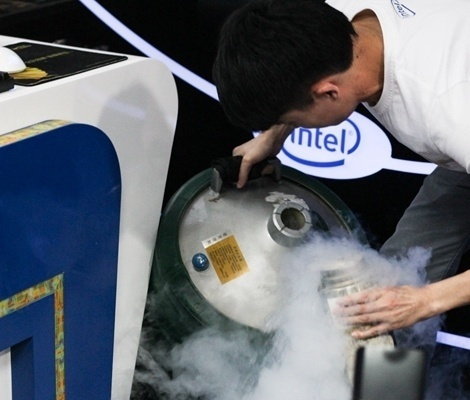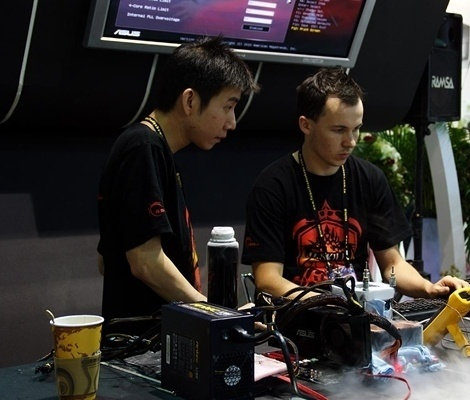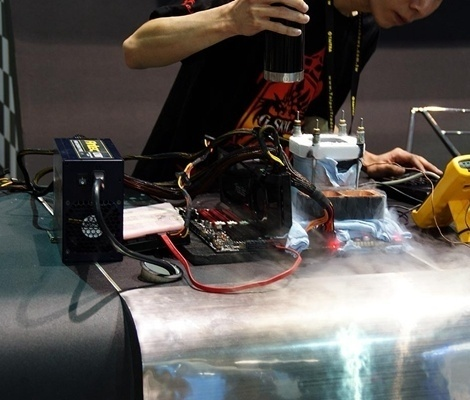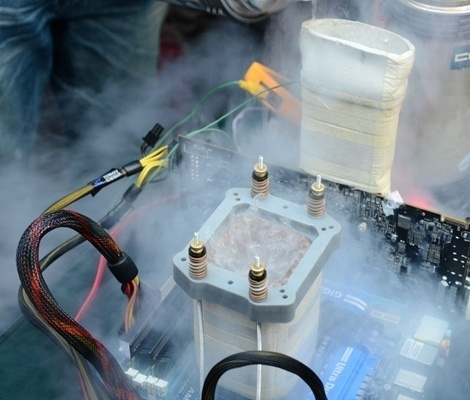|

# 液氮超频与普通超频的区别 有什么危害

随着计算机技术的发展，现在硬件的性能可是越来越强悍了，有很多发烧级别的玩家就开始玩起追求极致性能的游戏，不但要购买最新出品的，使用了最新技术的显卡和处理器，而且还使用各种技术进行超频，所谓的超频就是通过技术手段，让硬件在不属于原来的频率上工作，这种超频的手段能够让硬件在一定的时间内获得更高的性能，但是这种手段对于硬件本身来说，损害是无法避免的。

液氮超频与普通超频的区别

CPU在超频时会产生大量的热，普通超频一般指风冷或者水冷进行冷却，如果超的太多这两种冷却法就无法让CPU保持在一个适合的温度，造成失败，液氮温度很低，其他冷却可以比以上两种冷却法效果强很多，但是成本很高，由于液氮气化时吸热非常快，因此空气中的水蒸气将会凝结在铜管表面，所以必须在外面套一层绝缘橡胶材料，这种材料还必须要有保温作用，以防止液氮产生的“冷能”浪费，在超频过程中节省液氮用量!容器底部的铜底做成了蜂窝状，显然是为了增加液氮和铜块的接触面积，这样能够加速液氮的沸腾，达到迅速制冷的目的。

底座部分的防护工作也不容忽视，CPU附近的温度非常低，所以尽可能不让他与空气接触，防止冷凝产生的露水滴落在主板上产生悲剧事故!可能有些人觉得比较纳闷，这个蒸发皿就相当于一个散热器，那么散热器为什么没有扣具呢?是用液氮超频自然在开放环境下进行，这样的话只要将蒸发皿立在CPU上面就可以了，导热硅脂都不需要(好像还没有能禁受住-100℃低温的导热硅脂)，铜管自身的重量就能够很好的传热了。

云雾瞭绕中，CPU恍然置入仙境!液氮超低温的优势就在于可以给CPU加高压，“高压之下必有勇夫”，CPU/GPU的散热完全不用担心，因此可以尝试平时不敢奢想的高压，在高压下就能冲击更高频率。即便是普通状况下被定义为“不好超”的CPU，在液氮的推动下也能爆发出惊人的威力。

液氮超频有什么意义呢

抛去为了超频而超频的骨灰级玩家，玩家对显卡超频的原因只有一个：在XX游戏中，这片卡跑的“慢”，可调低分辨率、降低游戏效果，又失去了游戏的精髓。于是，在无法购买更高一级显卡的条件下，玩家只能通过内部挖潜，对显卡进行超频，进而提高其整体性能。对显卡而言，超频超的主要是GPU(显示核心)和显存。GPU是一片显卡的灵魂，直接决定了显卡的性能。它的速度可以通过提高GPU的工作频率来提高。而显存对显卡的重要性，仅次于核心，其类型和工作频率直接决定了显卡的带宽。我们可以把显示芯片想像成1辆时速300公里的“跑车”，只有在设计达到时速300公里以上的“高速公路“(显存带宽)上，才可以发挥出它的最大性能。所以，对显卡超频而言，核心和显存必须兼顾，单独对某一项进行超频都是没有实际意义的。

而液氮超频是超频中的一种极限，一来有挑战极限的意思，二来也可以追求更高的性能。硬件超频的危害性

所谓超频，实际上就是指让电脑的配件在高于标准频率下工作的一行为。其中主要包括CPU的超频，显卡的超频，以及内存的超频，而CPU的超频较早出现。

其实早期的超频行为实在是一种无奈，为了追求高效率，而那时的配件贵的出奇，无奈之下才超频应用的，而现在虽然有一少部分人是为了花较少的钱而达到较高的性能(其机器若不超频的话应用会有一定困难)，即追求性价比而超频的，其实更大一部分人纯粹就是一种跟风，别人说2500+能超到3200+，就去买2500+接着就去超，不知道那种人有没有想过，自己的2500+到底够不够用。还有一少部分人，那就是超频纯粹就是为了追求高数字，这种人也许不玩游戏，电脑很少干别的，进行最多的就是测试，对高性能的配件很感冒，这种人有一个共同的特点，那就是有钱!最后一类，是对超频有一定的兴趣，但是很少让电脑长时间的超频应用。仅仅是看看自己的电脑到底能不能超，我就是这种人。而高于标准频率工作，自然会对该硬件造成一定的损坏，有的损坏是缓慢的，有的则是瞬间的，你的CPU就可能Biu-的一下爆掉!似乎现在很多人对超频很感兴趣，因为超频能够让你的计算机获得更加高的性能，但是超频造成的后果是不可逆转的，但是适当的超频似乎能够让计算机的利用率更加高，比如说，在控制温度的超频中，能够让你的计算机的性能提高百分之三十，但却让你计算机的使用年限从二十年缩减到十年，但是一台电脑一般无法使用二十年之久，所以使用十年也差不多了，所以说适当超频也是可以的。

`声明：本文由入驻焦点开放平台的作者撰写，除焦点官方账号外，观点仅代表作者本人，不代表焦点立场错误信息举报电话： 400-099-0099，邮箱：jubao@vip.sohu.com，或点此进行意见反馈，或点此进行举报投诉。`A B C D E F G H J K L M N P Q R S T W X Y Z
A - B - C - D - E
• A
• 鞍山
• 安庆
• 安阳
• 安顺
• 安康
• 澳门
• B
• 北京
• 保定
• 包头
• 巴彦淖尔
• 本溪
• 蚌埠
• 亳州
• 滨州
• 北海
• 百色
• 巴中
• 毕节
• 保山
• 宝鸡
• 白银
• 巴州
• C
• 承德
• 沧州
• 长治
• 赤峰
• 朝阳
• 长春
• 常州
• 滁州
• 池州
• 长沙
• 常德
• 郴州
• 潮州
• 崇左
• 重庆
• 成都
• 楚雄
• 昌都
• 慈溪
• 常熟
• D
• 大同
• 大连
• 丹东
• 大庆
• 东营
• 德州
• 东莞
• 德阳
• 达州
• 大理
• 德宏
• 定西
• 儋州
• 东平
• E
• 鄂尔多斯
• 鄂州
• 恩施
F - G - H - I - J
• F
• 抚顺
• 阜新
• 阜阳
• 福州
• 抚州
• 佛山
• 防城港
• G
• 赣州
• 广州
• 桂林
• 贵港
• 广元
• 广安
• 贵阳
• 固原
• H
• 邯郸
• 衡水
• 呼和浩特
• 呼伦贝尔
• 葫芦岛
• 哈尔滨
• 黑河
• 淮安
• 杭州
• 湖州
• 合肥
• 淮南
• 淮北
• 黄山
• 菏泽
• 鹤壁
• 黄石
• 黄冈
• 衡阳
• 怀化
• 惠州
• 河源
• 贺州
• 河池
• 海口
• 红河
• 汉中
• 海东
• I
• J
• 晋中
• 锦州
• 吉林
• 鸡西
• 佳木斯
• 嘉兴
• 金华
• 景德镇
• 九江
• 吉安
• 济南
• 济宁
• 焦作
• 荆门
• 荆州
• 江门
• 揭阳
• 金昌
• 酒泉
• 嘉峪关
K - L - M - N - P
• K
• 开封
• 昆明
• 昆山
• L
• 廊坊
• 临汾
• 辽阳
• 连云港
• 丽水
• 六安
• 龙岩
• 莱芜
• 临沂
• 聊城
• 洛阳
• 漯河
• 娄底
• 柳州
• 来宾
• 泸州
• 乐山
• 六盘水
• 丽江
• 临沧
• 拉萨
• 林芝
• 兰州
• 陇南
• M
• 牡丹江
• 马鞍山
• 茂名
• 梅州
• 绵阳
• 眉山
• N
• 南京
• 南通
• 宁波
• 南平
• 宁德
• 南昌
• 南阳
• 南宁
• 内江
• 南充
• P
• 盘锦
• 莆田
• 平顶山
• 濮阳
• 攀枝花
• 普洱
• 平凉
Q - R - S - T - W
• Q
• 秦皇岛
• 齐齐哈尔
• 衢州
• 泉州
• 青岛
• 清远
• 钦州
• 黔南
• 曲靖
• 庆阳
• R
• 日照
• 日喀则
• S
• 石家庄
• 沈阳
• 双鸭山
• 绥化
• 上海
• 苏州
• 宿迁
• 绍兴
• 宿州
• 三明
• 上饶
• 三门峡
• 商丘
• 十堰
• 随州
• 邵阳
• 韶关
• 深圳
• 汕头
• 汕尾
• 三亚
• 三沙
• 遂宁
• 山南
• 商洛
• 石嘴山
• T
• 天津
• 唐山
• 太原
• 通辽
• 铁岭
• 泰州
• 台州
• 铜陵
• 泰安
• 铜仁
• 铜川
• 天水
• 天门
• W
• 乌海
• 乌兰察布
• 无锡
• 温州
• 芜湖
• 潍坊
• 威海
• 武汉
• 梧州
• 渭南
• 武威
• 吴忠
• 乌鲁木齐
X - Y - Z
• X
• 邢台
• 徐州
• 宣城
• 厦门
• 新乡
• 许昌
• 信阳
• 襄阳
• 孝感
• 咸宁
• 湘潭
• 湘西
• 西双版纳
• 西安
• 咸阳
• 西宁
• 仙桃
• 西昌
• Y
• 运城
• 营口
• 盐城
• 扬州
• 鹰潭
• 宜春
• 烟台
• 宜昌
• 岳阳
• 益阳
• 永州
• 阳江
• 云浮
• 玉林
• 宜宾
• 雅安
• 玉溪
• 延安
• 榆林
• 银川
• Z
• 张家口
• 镇江
• 舟山
• 漳州
• 淄博
• 枣庄
• 郑州
• 周口
• 驻马店
• 株洲
• 张家界
• 珠海
• 湛江
• 肇庆
• 中山
• 自贡
• 资阳
• 遵义
• 昭通
• 张掖
• 中卫

1室1厅1厨1卫1阳台

1
2
3
4
5

0
1
2

1

1

0
1
2
3报名成功，资料已提交审核A B C D E F G H J K L M N P Q R S T W X Y Z
A - B - C - D - E
• A
• 鞍山
• 安庆
• 安阳
• 安顺
• 安康
• 澳门
• B
• 北京
• 保定
• 包头
• 巴彦淖尔
• 本溪
• 蚌埠
• 亳州
• 滨州
• 北海
• 百色
• 巴中
• 毕节
• 保山
• 宝鸡
• 白银
• 巴州
• C
• 承德
• 沧州
• 长治
• 赤峰
• 朝阳
• 长春
• 常州
• 滁州
• 池州
• 长沙
• 常德
• 郴州
• 潮州
• 崇左
• 重庆
• 成都
• 楚雄
• 昌都
• 慈溪
• 常熟
• D
• 大同
• 大连
• 丹东
• 大庆
• 东营
• 德州
• 东莞
• 德阳
• 达州
• 大理
• 德宏
• 定西
• 儋州
• 东平
• E
• 鄂尔多斯
• 鄂州
• 恩施
F - G - H - I - J
• F
• 抚顺
• 阜新
• 阜阳
• 福州
• 抚州
• 佛山
• 防城港
• G
• 赣州
• 广州
• 桂林
• 贵港
• 广元
• 广安
• 贵阳
• 固原
• H
• 邯郸
• 衡水
• 呼和浩特
• 呼伦贝尔
• 葫芦岛
• 哈尔滨
• 黑河
• 淮安
• 杭州
• 湖州
• 合肥
• 淮南
• 淮北
• 黄山
• 菏泽
• 鹤壁
• 黄石
• 黄冈
• 衡阳
• 怀化
• 惠州
• 河源
• 贺州
• 河池
• 海口
• 红河
• 汉中
• 海东
• I
• J
• 晋中
• 锦州
• 吉林
• 鸡西
• 佳木斯
• 嘉兴
• 金华
• 景德镇
• 九江
• 吉安
• 济南
• 济宁
• 焦作
• 荆门
• 荆州
• 江门
• 揭阳
• 金昌
• 酒泉
• 嘉峪关
K - L - M - N - P
• K
• 开封
• 昆明
• 昆山
• L
• 廊坊
• 临汾
• 辽阳
• 连云港
• 丽水
• 六安
• 龙岩
• 莱芜
• 临沂
• 聊城
• 洛阳
• 漯河
• 娄底
• 柳州
• 来宾
• 泸州
• 乐山
• 六盘水
• 丽江
• 临沧
• 拉萨
• 林芝
• 兰州
• 陇南
• M
• 牡丹江
• 马鞍山
• 茂名
• 梅州
• 绵阳
• 眉山
• N
• 南京
• 南通
• 宁波
• 南平
• 宁德
• 南昌
• 南阳
• 南宁
• 内江
• 南充
• P
• 盘锦
• 莆田
• 平顶山
• 濮阳
• 攀枝花
• 普洱
• 平凉
Q - R - S - T - W
• Q
• 秦皇岛
• 齐齐哈尔
• 衢州
• 泉州
• 青岛
• 清远
• 钦州
• 黔南
• 曲靖
• 庆阳
• R
• 日照
• 日喀则
• S
• 石家庄
• 沈阳
• 双鸭山
• 绥化
• 上海
• 苏州
• 宿迁
• 绍兴
• 宿州
• 三明
• 上饶
• 三门峡
• 商丘
• 十堰
• 随州
• 邵阳
• 韶关
• 深圳
• 汕头
• 汕尾
• 三亚
• 三沙
• 遂宁
• 山南
• 商洛
• 石嘴山
• T
• 天津
• 唐山
• 太原
• 通辽
• 铁岭
• 泰州
• 台州
• 铜陵
• 泰安
• 铜仁
• 铜川
• 天水
• 天门
• W
• 乌海
• 乌兰察布
• 无锡
• 温州
• 芜湖
• 潍坊
• 威海
• 武汉
• 梧州
• 渭南
• 武威
• 吴忠
• 乌鲁木齐
X - Y - Z
• X
• 邢台
• 徐州
• 宣城
• 厦门
• 新乡
• 许昌
• 信阳
• 襄阳
• 孝感
• 咸宁
• 湘潭
• 湘西
• 西双版纳
• 西安
• 咸阳
• 西宁
• 仙桃
• 西昌
• Y
• 运城
• 营口
• 盐城
• 扬州
• 鹰潭
• 宜春
• 烟台
• 宜昌
• 岳阳
• 益阳
• 永州
• 阳江
• 云浮
• 玉林
• 宜宾
• 雅安
• 玉溪
• 延安
• 榆林
• 银川
• Z
• 张家口
• 镇江
• 舟山
• 漳州
• 淄博
• 枣庄
• 郑州
• 周口
• 驻马店
• 株洲
• 张家界
• 珠海
• 湛江
• 肇庆
• 中山
• 自贡
• 资阳
• 遵义
• 昭通
• 张掖
• 中卫• 手机• 分享
• 设计
免费设计
• 计算器
装修计算器
• 入驻
合作入驻
• 联系
联系我们
• 置顶
返回顶部